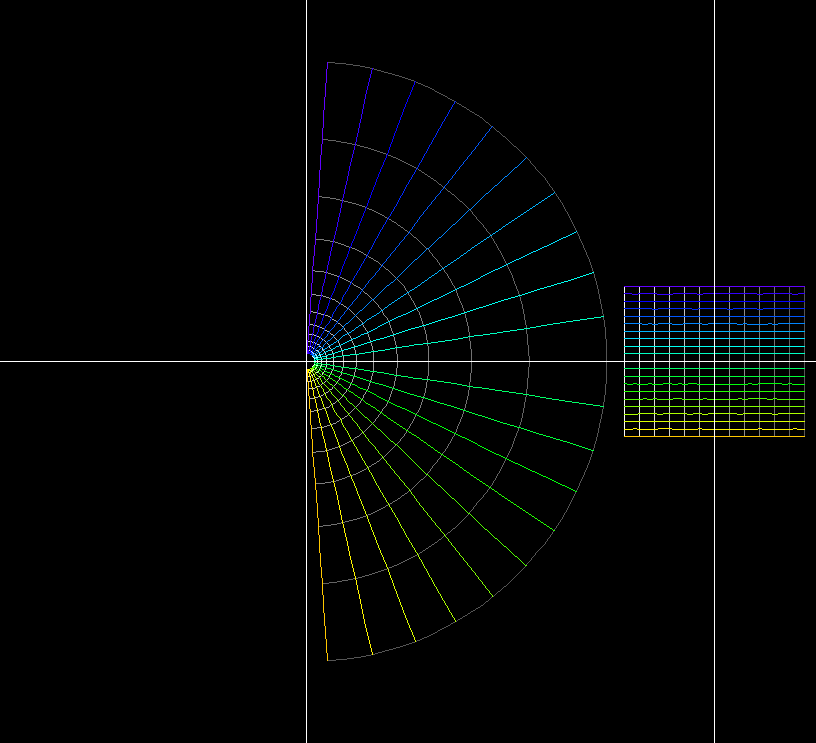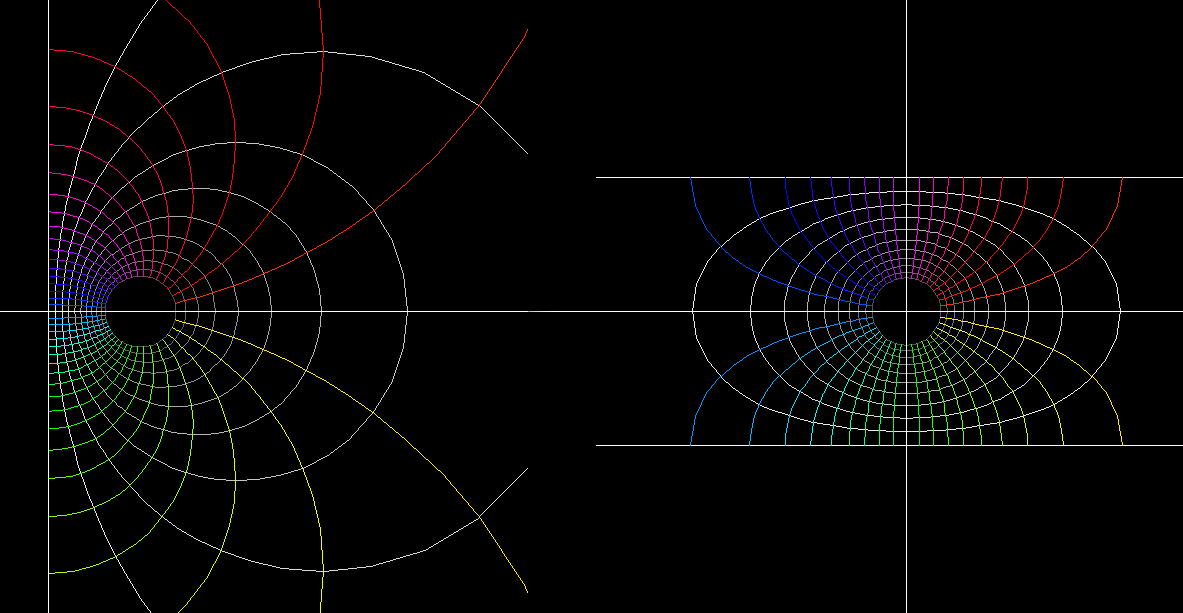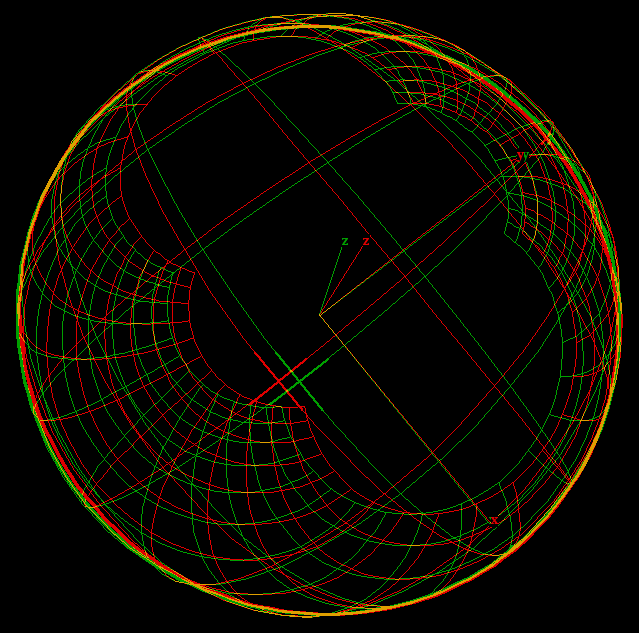# Log Function

The function `f(z) = log(z)` is an inverse of the exponential function: `log(exp(z)) = z`. But log(z) cannot be defined as a differentiable function in the full plane for two reasons:

1. log(0) does not exist because exp(z) is never 0. In other words, there is no number z which could serve as the logarithm of zero.
2. `exp(z)` is periodic, namely `exp(z) = exp(z + 2i*pi)`. This says that there are infinitely many choices for defining the logarithm of `w = exp(z)`, namely `log(w) = z, = z + 2i*pi, = z + 4i*pi …. , log(w) = z + n*2i*pi`, n integer.

The “principal value” of the complex logarithm is a differentiable function defined on the plane minus the negative real axis. The values of this function lie in the horizontal strip { z ; -pi < Im(z) < +pi }.

Nothing special happens on the negative real axis. One can define the complex logarithm as a differentiable function on the plane minus any half line from zero to infinity. The animation shows some choices.The left is pre-image. The right is the image of log(z)

`log(z)` is the inverse function of `exp(z)`. [see Complex Exponential Function]

exp is a periodic function, log cannot be defined as a differentiable function on the whole plane. The “principal value of log” is a differentiable function in the plane minus the negative real axis. The other values of log differ from the principal value by an integer multiple of 2 π. Since every z ≠ 0 has multiple logarithm values, the complex logarithm is called a “multivalued” function.The left is a pre-image. It is the image of the Moebius transformation 「z ⟶ (z-1)/(z+1)」. The right in the image of 「log(z)」. The result is an image that consists of polar coordinates in infinite strips. Note that the logarithm maps the right half plane to an infinite strip of wids pi.log1 polar Riemannlog2 polar RiemannAnna

z_log_.pdf